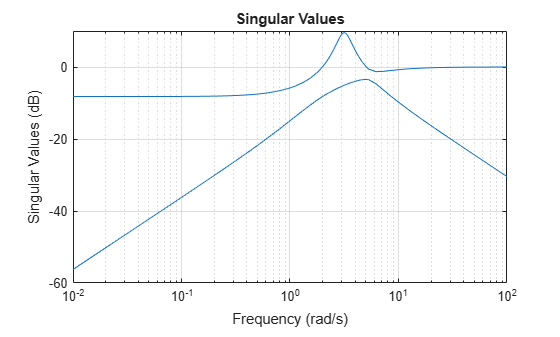hinfnorm

H norm of dynamic system

Description

ninf = hinfnorm(sys) returns the H norm in absolute units of the dynamic system model, sys.

• If sys is a stable SISO system, then the H norm is the peak gain, the largest value of the frequency response magnitude.

• If sys is a stable MIMO system, then the H norm is the largest singular value across frequencies.

• If sys is an unstable system, then the H norm is defined as Inf.

• If sys is a model that has tunable or uncertain parameters, then hinfnorm evaluates the H norm at the current or nominal value of sys.

• If is a model array, then hinfnorm returns an array of the same size as sys, where ninf(k) = hinfnorm(sys(:,:,k)) .

For stable systems, hinfnorm(sys) is the same as getPeakGain(sys).

ninf = hinfnorm(sys,tol) returns the H norm of sys with relative accuracy tol.

example

[ninf,fpeak] = hinfnorm(___) also returns the frequency, fpeak, at which the peak gain or largest singular value occurs. You can use this syntax with any of the input arguments in previous syntaxes. If sys is unstable, then fpeak = Inf.

Examples

collapse all

Compute the ${H}_{\infty }$ norm of the following 2-input, 2-output dynamic system and the frequency at which the peak singular value occurs.

$\mathit{G}\left(\mathit{s}\right)=\left[\begin{array}{cc}0& \frac{3\mathit{s}}{{\mathit{s}}^{2}+\mathit{s}+10}\\ \frac{\mathit{s}+1}{\mathit{s}+5}& \frac{2}{\mathit{s}+6}\end{array}\right].$

G = [0 tf([3 0],[1 1 10]);tf([1 1],[1 5]),tf(2,[1 6])];
[ninf,fpeak] = hinfnorm(G)
ninf = 3.0150
fpeak = 3.1623

The ${H}_{\infty }$ norm of a MIMO system is its maximum singular value. Plot the singular values of G and compare the result from hinfnorm.

sigma(G),gridThe values ninf and fpeak are consistent with the singular value plot, which displays the values in dB.

Input Arguments

collapse all

Input dynamic system, specified as any dynamic system model or model array. sys can be SISO or MIMO.

Relative accuracy of the peak gain, specified as a positive real scalar value. hinfnorm calculates ninf such that the fractional difference between ninf and the true H norm of sys is no greater than tol.

Output Arguments

collapse all

H norm of sys, returned as Inf, a scalar value, or an array.

• If sys is a single stable model, then ninf is a scalar value.

• If sys is a single unstable model, then ninf is Inf.

• If sys is a model array, then ninf is an array of the same size as sys, where ninf(k) = hinfnorm(sys(:,:,k)).

Frequency at which the peak gain or largest singular value occurs, returned as Inf, a nonnegative real scalar value, or an array. The frequency is expressed in units of rad/TimeUnit, relative to the TimeUnit property of sys.

• If sys is a single stable model, then fpeak is a scalar.

• If sys is a single unstable model, then fpeak is Inf.

• If sys is a model array, then fpeak is an array of the same size as sys.In this case, fpeak(k) is the peak gain or largest singular value frequency of the kth model in the array.